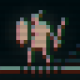# The Resolver In Depth

Included below are snippets of the main body of the solver.  Using two for loops that surround the main body of code, we can traverse all of the tiles that are overlapped by the object being tested. {x1, x2, y1, y2} are used as the limits in the for loops, with {x1,y1} being the upper left extreme tile overlapped of the object being tested, and {x2,y2} being the lower left tile.  The first step taken inside the loop is to index the tile map data and pull out the 8bit value that represent the current tile at [x,y] in tile space.  Each tile in my game is an encoding of bits that specify that tiles properties.  You can see that { cWall, cQUpper, cQLower, cQLeft, cQRight } are all bit masks that can be tested against a tile. The bit mask encoding is defined as this:

```// useful macro for encoding single bits
#define BIT( X ) (1<<(X))

// map bit codes
const uint8 cQUpper  = BIT( 0 ); // can push up
const uint8 cQLower  = BIT( 1 ); // can push down
const uint8 cQLeft   = BIT( 2 ); // can push left
const uint8 cQRight  = BIT( 3 ); // can push right
const uint8 cWall    = BIT( 7 ); // is a wall```

In the main loop, we can quickly skip over any tiles that are not walls since they don’t contribute in any way to the collision, or a resolution.  There are four directions in which a tile may push an object so as to resolve a collision, being { up, down, left, right }.  In the pre-processing step I have determined which directions are valid for each tile to push, and are encoded for each tile using the scheme above.  So, for each direction the tile can push, we compute a resolution vector that would safely push the overlapping object out of a collision with this tile.  These are stored in the array named ‘quad’, standing for quadrant.  After this step we have an array potentially full of four different resolution values.  A key point to note is that for each tile in the world, we limit it so that it may only push the object in one direction, even if it overlaps on multiple axes.  Therefore a method is needed to decide which axis is best to choose as our resolution.  This is easy in fact, as it is simply that which would move the object by the smallest amount.  The ‘select_from_absmin()’ function is simple but usefull, it simply returns the argument who has the smallest absolute (forced positive) value.  We start off finding the best resolution for each of the two axes, x and y.  Next we challenge these two, deciding if the x axis has a easier resolution then that on the y axis.  Outside of this loop we are maintaining two variables that store the final resolution for each axis, dp_x and dp_y, standing for displacement_x, etc.  If the tile we have examined provides an easier resolution, or a smaller displacement, then we have currently in our dp_?, then we can take the new displacement as our new dp_?.

```// traverse all tiles in the collision area
for ( int y=y1; y<=y2; y++ )
for ( int x=x1; x<=x2; x++ )
{
// extract this tiles value
uint8 tile = map->data[ x + y*map->width ];

// skip if not a blocking tile
if ( (tile & cWall) == 0 )
continue;

// four resolution values, one for each resolution direction
int quad = { 0xFFFF, 0xFFFF, 0xFFFF, 0xFFFF };

// find all of the resolutions, one for each direction
if ( (tile & cQUpper) != 0 ) quad =  (y   *ts) - info->y2;
if ( (tile & cQLower) != 0 ) quad = ((y+1)*ts) - info->y1;
if ( (tile & cQLeft ) != 0 ) quad =  (x   *ts) - info->x2;
if ( (tile & cQRight) != 0 ) quad = ((x+1)*ts) - info->x1;

// select smallest resolution for each axis by comparison of their abs min

// select the smallest resolving axis for this tile
{
// x - axis
// save it if smaller then our current resolution
if ( absi( quad ) < absi( dp_x ) ) dp_x = quad;
}
else
{
// y - axis
// save it if smaller then our current resolution
if ( absi( quad ) < absi( dp_y ) ) dp_y = quad;
}
}```

By the end of this process we may have two displacement values for each axis, dp_x and dp_y, and they can simply be returned to the calling function.  The algorithm itself is quite simple and easy to visualize when you are familiar with it.  It shows good robustness when given small interpenetration depths, of perhaps 1/3 of a tile size.  In reality this is quite acceptable, since it would be rare to suddenly have a character in a game penetrate the world geometry beyond that much.  Only at extreme velocities would we penetrate more then this amount, but then we can perform actions to guard against it, such as testing for collisions over fixed distances, so add half of the velocity and test, then the second half and test again.  Essentially that would be a subdivision scheme.  In other situations we can perform ray casts to instantly compute a collision location for very fast objects.

Code for the full solver can be read here:

collide.h

collide.cpp